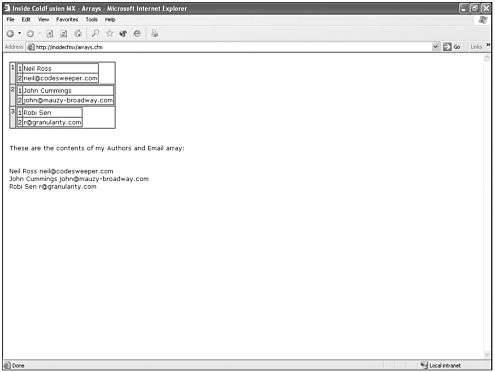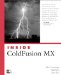# Arrays

An array is an arrangement of memory elements in one or more planes. A ColdFusion array object stores indexed values. Each element in the array has a number assigned to it that is referred to as its index. ColdFusion arrays can have one or more dimensions or planes.

Arrays with a single dimension resemble a column of values (see Figure 7.5). Multidimensional arrays resemble a complex spreadsheet (see Figure 7.6).

##### Figure 7.5. One-dimensional array.##### Figure 7.6. Multidimensional array.You'll see the same kind of layout for your arrays when you use the CFDUMP tag to view their contents.

You should remember the following about ColdFusion arrays:

• ColdFusion arrays are limited to three dimensions.

• ColdFusion array indexes start at 1.

• ColdFusion array sizes can be reset after creation.

• Elements can be added or deleted from ColdFusion arrays.

#### Creating an Array

When you create an array within ColdFusion code, you are "initializing" the array. This is a common term in computer programming. To initialize an array in ColdFusion, you use the ArrayNew() function, as shown in the following code:

` <cfset a_Authors=ArrayNew(1)> `

The ArrayNew() function takes a single argument: a numeric value that sets the dimensions of the new array. As such, the example in the preceding code snippet would initialize a new array with a single dimension. In contrast, the following code snippets initialize two- and three-dimensional arrays, respectively:

` <cfset a_AuthorAndEmail=ArrayNew(2)>  <cfset a_AuthorData=ArrayNew(3)>  `

#### Adding Elements to an Array

When you initialize your ColdFusion array, it contains no data. You must fill the array with useful bits of information. One way to add elements to an array is with the simple and familiar CFSET call:

` <cfset a_Authors="Neil Ross">  <cfset a_Authors="John Cummings">  <cfset a_Authors="Robi Sen"> `

Another way to handle the addition of elements and values to your array is to use the ArrayAppend() function. The ArrayAppend() function inserts a new value at the end of the array. The basic form of the ArrayAppend() function call looks like this:

` ArrayAppend(arrayname, "new value") `

Examine the following code:

` <cfset tempvar=ArrayAppend(a_Authors, "Just Kidding")> `

As we add more and more information to the array, it expands to accept the new data. You should always start filling your array with the first element element 1 and continue without leaving gaps. ColdFusion sees gaps as undefined array elements and might throw errors in your application. In addition, you can do any sorting of the data in your array later. Adding elements to multidimensional arrays is not much different:

` <cfset a_AuthorAndEmail="Neil Ross">  <cfset a_AuthorAndEmail="neil@codesweeper.com">  <cfset a_AuthorAndEmail="John Cummings">  <cfset a_AuthorAndEmail="john@mauzy-broadway.com">  <cfset a_AuthorAndEmail="Robi Sen">  <cfset a_AuthorAndEmail="r@granularity.com"> `

Note

You cannot append elements and values to a multidimensional array using the ArrayAppend() function.

Before we move headlong into creating output loops for array contents, take a moment to verify that the contents of your array are as you expect them to be. In addition, you should inspect the array structure by calling the CFDUMP tag, as shown in the following code:

` <cfdump var="#a_AuthorAndEmail#"> `

#### Outputting an Array

You can output the values held in an array by creating a loop over each element in that array. You use, of course, the CFLOOP and CFOUTPUT tags in combination to output the called variables in a position that corresponds to each loop index.

Let's begin with an easy example. First, working with the a_Authors array, you can create a loop for each element in the array with CFLOOP. You call the CFOUTPUT tag within the CFLOOP tag itself. The result is that CFOUTPUT is performed once for each item in the array, based on the index number of the loop. In an index loop, the index of the loop represents a whole number that correlates to the loop count:

` <cfloop index="i" from="1" to="#ArrayLen(a_Authors)#">           <cfoutput>#a_Authors[i]#<br></cfoutput>  </cfloop> `

Now let's take a look at the preceding code in action (see Listing 7.6).

##### Listing 7.6 Create the a_Authors Array and the Output Loop
` <html>  <head>          <title>Inside ColdFusion MX - Arrays</title>  </head>  <body>  <table>       <tr>            <td>                 <cfsetting enablecfoutputonly="Yes">                      <cfset a_AuthorAndEmail=ArrayNew(2)>                      <cfset a_AuthorAndEmail="Neil Ross">                      <cfset a_AuthorAndEmail="neil@codesweeper.com">                      <cfset a_AuthorAndEmail="John Cummings">                      <cfset a_AuthorAndEmail="john@mauzy-broadway.com">                      <cfset a_AuthorAndEmail="Robi Sen">                      <cfset a_AuthorAndEmail="r@granularity.com">                 <cfsetting enablecfoutputonly="No">                 These are the contents of my Authors and Email array:                 <br>                 <br>                 <cfloop index="author" from="1" to="#ArrayLen(a_AuthorAndEmail)#">                       <cfloop index="email" from="1"  to="#ArrayLen(a_AuthorAndEmail[author])#">                           <cfoutput>#a_AuthorAndEmail[author][email]#</cfoutput>                      </cfloop><br>                 </cfloop>            </td>       </tr>  </table>  </body>  </html> `

The output should appear as shown in Figure 7.7.

##### Figure 7.7. Simple display of the contents of the a_Authors array.Outputting a two-dimensional array is a bit different, but only in respect to how the second dimension is handled. To output the elements from both dimensions, you use two CFLOOP tags. One is nested within the other and the CFOUTPUT tag is inside the inner loop. Don't let it scare you though. It's this simple:

` <cfloop>        <cfloop>              <cfoutput></cfoutput>        </cfloop>  </cfloop> `

Of course, you can also output a three-dimensional array. The technique that we've used for the one- and two-dimensional arrays would be followed with an additional nested CFLOOP.

#### Array Functions

As with queries and lists, ColdFusion has different native functions that enable you to manipulate arrays from sorting to clearing to resizing. The following array functions are available to ColdFusion developers:

 ArrayAppend ArrayIsEmpty ArrayPrepend ArraySwap ArrayAvg ArrayLen ArrayResize ArrayToList ArrayClear ArrayMax ArraySet IsArray ArrayDeleteAt ArrayMin ArraySort ListToArray ArrayInsertAt ArrayNew ArraySum

` <cfset tmp=ArrayPrepend(a_Authors, "Just Kidding")> `

• IsArray(). Checks whether the variable is an array and returns Yes or No :

` <cfset tmp=IsArray(a_Authors)> `

• ArrayDeleteAt(). Deletes the specified element in the array and shifts the remaining array elements to fill the deleted index:

` <cfset tmp=ArrayDeleteAt(a_Authors, 3)> `

• ArrayClear(). Removes all the data from the array:

` <cfset tmp=ArrayClear(a_Authors)>  `Inside Coldfusion MX
ISBN: 0735713049
EAN: 2147483647
Year: 2005
Pages: 579